Rayleigh Waves: Exploring the Surface

George Karakonstantakis March 20, 2008

(Submitted as coursework for Physics 372, Stanford University, Winter 2008)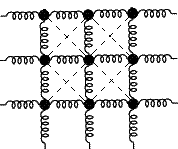Fig. 1: Illustration of 2d ball and spring model showing 1d surface.

Problems with masses and springs are widespread in physics literature. The reason for this, being their mathematical simplicity, the clarity of their results and, most importantly, the fact that they present excellent prototype models for many physical problems . For these reasons we are to study a very simple two-dimensional model of masses attached to springs. The masses are placed in a square lattice and they are connected with their nearest and next nearest neighbors with springs. We will investigate, in this very simple system, the response of the masses at the surface (and we will compare them with that of the masses of the interior) namely, the oscillations or waves that exist and travel on the surface. Doing this we will discover the existence of the Rayleigh waves (waves that they live and travel in the surface) and we will gain some insight of their properties and dynamics.

Classical Springs on a Lattice and Response Runction

The model that we are to use is a very simple and, at the same time, very pedagogical. It is illustrated in Fig. 1. We will consider a square lattice of bond length b which consists of masses connected together with springs as shown in the figure above. There are two types of springs in our model. The first type are springs that connect nearest neighbor masses, having spring constant k1 which are denoted by curly lines. The other type, denoted by broken lines, are springs that connect the next nearest neighbors, having different spring constant k2. This second set of springs might give the impression that it makes our problem much more complicated, but it does not. In a matter of fact, it is only going to make the equations a little longer. Had it not been for this second type of springs though, the behavior of the surface would have been just trivial.

Having all these thing in mind, we can easily write down the equations of motion that this model obeys. But before we do this, let’s make a couple of comments and assumptions that they will make this task simpler. We will consider the lattice described above to be semi-infinite in the y-direction - that is infinite in the negative y-direction but has a bound in the positive one - so that our system has a surface to investigate. Furthermore we will impose periodic boundary conditions in the x-direction, which means that we are to close every chain in x-axis to itself. This will simplify our problem considerably. The periodic boundary conditions make the vibration of one mass to be the same as that of its neighbor-mass (in the x-direction) times eiqb, q being the x component of the wave vector. This makes our problem from a two dimensional to a quasi-one dimensional problem, which is much easier. Thus, we will write the equations of motion for the masses of every y-chain with the following enumeration: the surface mass will be tagged by n=0 and as we go down to the y-direction n increases. Last but not least, we will set all the masses and the bond length equal to 1.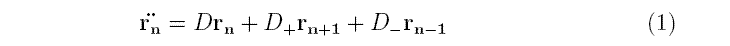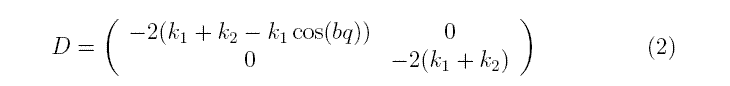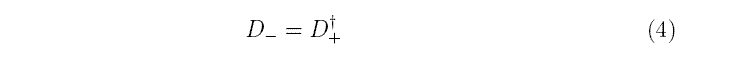These are the equations of motion for our system. The matrix D (the so called dynamical matrix) gives the contribution to the force due to the motion of the nth mass. The matrix D+ gives the contribution due to the motion of the mass n+1 and D- that of mass n-1. Now we can use these equations for the Green's function as follows: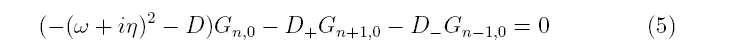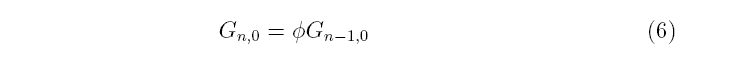Now Green's function is the one that it will give the information about the response of both the interior (the bulk as it is commonly called) and the surface of the system. That is why we will call, from now on, the imaginary part of the Green's function, response function. Now it is very easy to solve the Eq. (5) taking into account Eq. (6). So if we apply Eq. (6) to Eq. (5) we will get the results: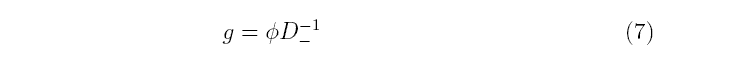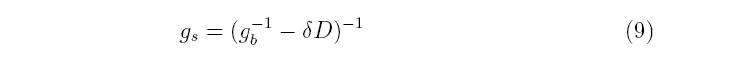Eq. (8) being the response of the bulk while Eq. (9) is the response of the surface. This is difference is, of course, due to the fact that the masses at the surface are connected to fewer springs that the ones in the bulk. This is the origin of the term δ D , the change in the dynamical matrix that we must do to get the response of the surface.

We have solved these equations by a little Fortran 77 program which you can find here. In order for us to solve the problem we used k1=1 and k2=0.5.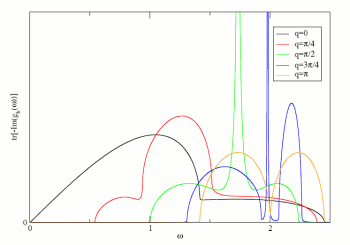Fig. 2: Response in the bulk.

Results and Discussion

With our program, mentioned above, we calculated the response function for our system. First of all the response of the interior (or the bulk as it is also known) of the system was calculated so that we know the spectrum of the vibrations there. Then the response of the surface was calculated in the way that we described above. These results are plotted (for different values of q)

The response graphs can be understood in the following, very simple, way. Wherever we have non-zero value of the response function, we can have oscillations with this frequency. On the other hand, if we have zero value of the response function we cannot have any oscillation (or wave in general) with this frequency. On the light of these remarks we can now look, explain and interpret the results, starting from the bulk.

The very first thing that we observe in this spectrum is the presence of a gap in the allowed vibration modes that is developing with increasing q. This means that for a given non-zero q there is a frequency below which we have no vibrations (this is often referred to as cut-off frequency). So a wave or a collective oscillation with frequency less than the cut-off frequency just cannot propagate in the bulk. Another thing that we have to mention is that we have averaged over the two different ways that the masses can oscillate i.e vertically or horizontally. We did that via taking the trace of the response function. Other than that there is nothing special about that spectrum. It is pretty straight-forward and it does not have any strange features. Now let's move to the, more interesting, response of the surface.

We can clearly see that there is a peak, for every non-zero q, that appears inside the gap that we were talking about previously, just next to the cut-off frequency. This is the so called Rayleigh wave, a wave that can exist only in the surface. We can easily understand why this is so. The Rayleigh peak is located inside the gap, of the bulk response function. This means that it cannot propagate in the bulk of our system and, therefore, it has to decay evanescently. Another point, which cannot see it in the graph, is that this wave contains both of the two different polarizations (transverse and longitudinal). This cannot be seen in our graph because we have averaged over these two polarizations, but it can be checked by plotting the diagonal components of the response separately. The most important feature of these waves, though, is that they are model-independent. This means that they will show up in any model we might choose, provided that we will include next-nearest neighbor interactions in it.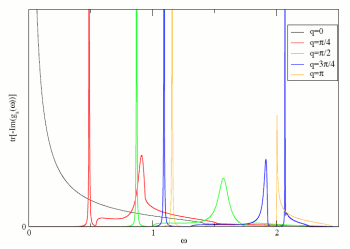Fig. 3: Response at the surface.

These kinds of waves are of outmost importance in several domains in science. First and foremost they are earthquake waves so their properties and dynamics have been studied extensively and they are very well understood from geologists and seismologists, because they are the most destructive waves in an earthquake. Furthermore they are very important to physics too, because they are a very important part of the surface dynamics of materials and because we can directly measure them from experiments. There are experiments like the one described in ref  of Helium atom scattering, in which the cross sections measured are directly proportional to the surface response function.

Concluding Remarks

To sum up, we have investigated the behavior of a very simple model of harmonic oscillators and we arrived at the conclusion that this possesses a very interesting range of oscillations at the surface, the so called Rayleigh waves. Moreover we have seen here that even a very simple model, with very simple manipulations and calculations can yield to non-trivial results, which are of utmost importance.

© 2008 George Karakonstantakis. The author grants permission to copy, distribute and display this work in unaltered form, with attribution to the author, for noncommercial purposes only. All other rights, including commercial Rights are reserved to the author.

References

 P. C. Martin, Measurements And Correlation Functions (Gordon and Breach, 1968).

 I. A. Viktorov, Rayleigh and Lamb Waves (Plenum, 1967).

 J. P. Toennies et al., Phys. Rev. B 65, 165427 (2002).